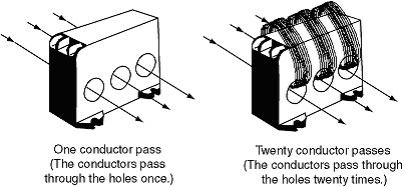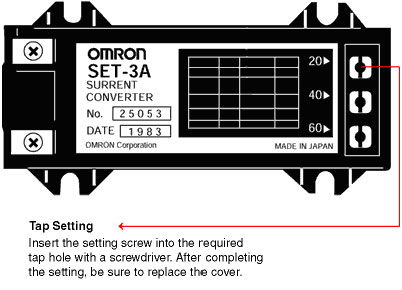# Can SE Motor Protective Relays be used for motors with a rated current of 1 A or less?

Yes, you can do this by using the SET-3A Current Converters. Set the primary conductor runs to 20 passes, and set the tap setting to 20.

*The current scale multiplying factor will be 0.1.

Example: Using the SET-3A and a Tap Setting of 20

Reference

 Rated current(stable current range) (A) Current Converter Passes Tap setting Model 0.4 to 1 20 20 SET-3A 0.8 to 2 10 20 4 to 10 2 20 8 to 20 1 20

Note:(Tap setting)/ (Passes) = Maximum scale (10) value

Interpreting the Above Table:

In the darkly shaded row, the rated current range can be set to 0.4 to 1 A with 20 passes. This can be used for motors of 1 A or less. Refer to the following explanations to determine the number of primary conductor runs (passes) and the tap setting.

Determining the Number of Primary Conductor Passes

Pass all three wires through the holes from the same direction. It doesn't matter which wires go through which holes.# Did this help answer your question?Thanks for the feedback! 🙏🏽